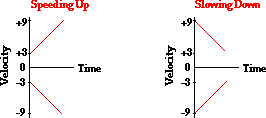# Going over my first exam (Kinematics)

Chandasouk
I took my first hourly a couple of weeks ago and barely passed it. I was wondering if someone could go over the questions I got wrong since this is going to haunt me in the future?

I. An 8kg crate is being pulled up with a force of F_pull = 60N. Assume the rope is massless and cannot be stretched.

C) The rope can withstand a max tension of 85N without breaking. What is the largest upward acceleration that the crate can experience without the rope breaking?

I did not know how to do this problem. Would I do like Fnet = 85N - 78.4N = 6.6NN

and F=ma

6.6N = 8kga

a = 0.825m/s^2 ?

I'm really not sure.

Problem #4

A child is standing on a bridge throws a rock straight down. The rock leaves the child's hand at t=0. Which of the graphs shown here best represents the velocity as a function of time?The first graph on the bottom left , but I do not understand why. Originally, I selected a graph that resembled the first graph on the bottom left, but it's line started at the origin.

Problem #5

A rock is thrown directly upward from the edge of the roof of a building that is 34.6m tall. The rock misses the building on its way down and is observed to strike the ground 4.0 seconds after being thrown. With what speed was the rock thrown?

I had no clue how to do this and my answer i selected was wrong. What I did was

34.6m = vi(4s) + 1/2(-9.8m/s^2)(4s)2

vi=28.25m/s which was incorrect. The real answer was 11m/s.

An index to describe motion: A bicyclist travels in the negative direction. You measure his velocity at several instants in time, and plot the data. You find that the velocity versus time graph is linear, with a slope of +2.2.

Use everyday language to describe the info the slope tells about this situation. Specifically what does 2.2 mean and what does the "+" mean?

Last edited:

highcontrast
I will give it an attempt, but I'm still new to physics. So, if my answers are errenous, please, anyone, feel free to correct them.

for 1) it's important to realize for an upward accleration, the nforce acting upwards must be greater than the accleration force.
So, the accleration force acting on the box is 78.4N.
Since the rope will break > 85 Newtons, 85N for the rope.
Since n>g, 85-78.4 = 6.6N
Accleration = Fnet/mass = 6.6N/8 = 0.825m/s^2

look416
s1=ut1+$$\frac{1}{2}$$x(-9.8)t12
s2=$$\frac{1}{2}$$x(9.8)t22
t1+t2=4s
so, t2=4-t1
s2=34.6m
so, 34.6=$$\frac{1}{2}$$x(9.8)xt22
Now substitue t2=4-t1 into the equation
34.6=$$\frac{1}{2}$$x9.8x(4-t1)2
34.6=$$\frac{1}{2}$$x9.8x(t12-8t1+16)
now solve the equation
69.2=9.8t12-78.4t1+156.8
9.8t12-78.4t1-87.6=0
t1=